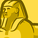# QlikView App Dev

Discussion Board for collaboration related to QlikView App Development.

Announcements
Action-Packed Learning Awaits! QlikWorld 2023. April 17 - 20 in Las Vegas: REGISTER NOW
cancel
Showing results for
Did you mean:Creator II

## Straight Table row data in Text Box

I have a straight table with Dimension as

Dim: =If(Aggr(Rank(Count(DISTINCT{\$<Type={'Sale'}>}(ID))) <= 5,EID),EID)

Expression: Count(DISTINCT{\$<Type={'Sale'}>}(ID))

This is to show only top 5 EIDs in the Straight Table that has the most number of IDs

How do I show the top most EID and number of IDs this EID has in a Tex Box?

Labels (3)

• ### Other

1 Solution

Accepted SolutionsMVP

May be try like below

=FirstSortedValue(EID, aggr(rank(Count(DISTINCT{\$<Type={'Sale'}>}ID),4),EID))

which gives Top most EID info.

Or

=aggr(if(Rank(Count(DISTINCT{\$<Type={'Sale'}>}ID))=1, EID, EID)

------------

Below exp. gives the number of IDs of that EID

Aggr(If(rank(Count(DISTINCT{\$<Type={'Sale'}>}ID),4) = 1, Count(DISTINCT{\$<Type={'Sale'}>}ID)),EID)

Thanks & Regards,
Mayil Vahanan R
4 RepliesMVP

May be try like below

=FirstSortedValue(EID, aggr(rank(Count(DISTINCT{\$<Type={'Sale'}>}ID),4),EID))

which gives Top most EID info.

Or

=aggr(if(Rank(Count(DISTINCT{\$<Type={'Sale'}>}ID))=1, EID, EID)

------------

Below exp. gives the number of IDs of that EID

Aggr(If(rank(Count(DISTINCT{\$<Type={'Sale'}>}ID),4) = 1, Count(DISTINCT{\$<Type={'Sale'}>}ID)),EID)

Thanks & Regards,
Mayil Vahanan RCreator II
Author

Works as expected. Thank you very much.Creator II
Author

Just like FirstSortedValue, do we have anything to show Top 2nd, Top 3rd etc similar to what we show using FirstSortedValue?MVP

Hi @qlikwiz123 ,

You can use rank () function or in firstsortedvalue function itself, u can provide rank..